# The Equal Tempered Octave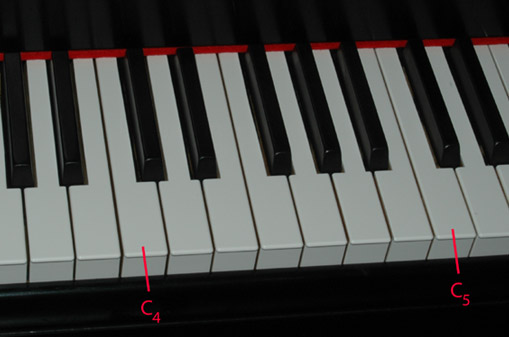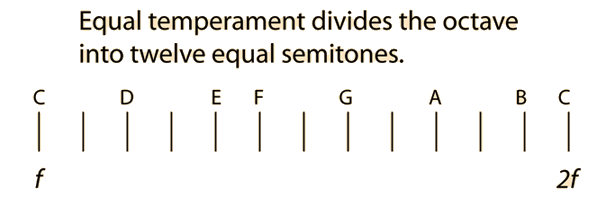The middle octave on the piano is shown as a standard example of equal temperament. Since a musical interval is defined by a ratio, the division of an octave into 12 equal intervals (equal tempered semitones) involves finding the ratio by which you multiply the starting frequency f twelve times to get a frequency 2f. If this ratio is represented by a, then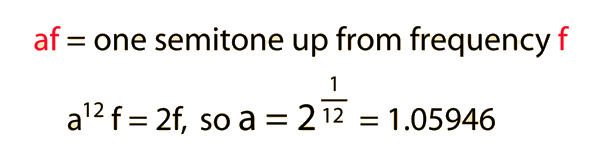Proceeding up the equal tempered scale, each note is about 6% higher than the previous note.

 Equal tempered octave vs other temperaments
 Equal tempered frequency list
Index

Temperament and musical scales

 HyperPhysics***** Sound R Nave
Go Back

# Musical Clefs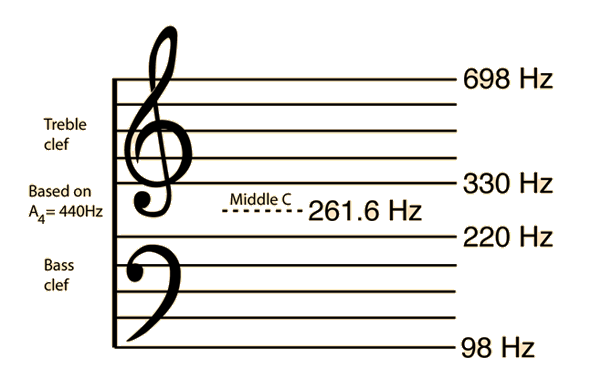Equal tempered frequency list
Index

Temperament and musical scales

 HyperPhysics***** Sound R Nave
Go Back

# Semitones Compared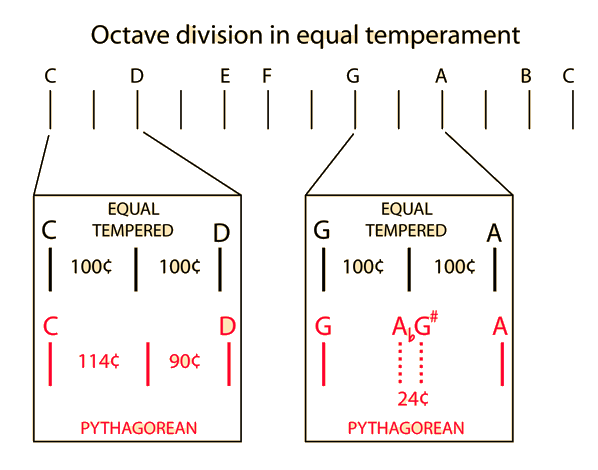The differences shown between semitones in Pythagorean and Equal Temperament are representative of the classic difficulties encountered in the building up of musical scales. Pythagorean temperament has two different sized semitones which lead to differences in chromatic notes like A-flat and G-sharp. This means that you cannot freely change keys for a piece of music written for Pythagorean temperament. In equal temperament, A-flat and G-sharp are the same black key on the piano, so that you can freely transpose between keys. On the other hand, that convenience comes at the price of deviations from the small-whole-number ratio rule for consonance.

 Pythagorean intervals
Index

Temperament and musical scales

Reference
Rossing
Science of Sound, 2nd ed.
Ch 7

 HyperPhysics***** Sound R Nave
Go Back

# Comparison of Musical Intervals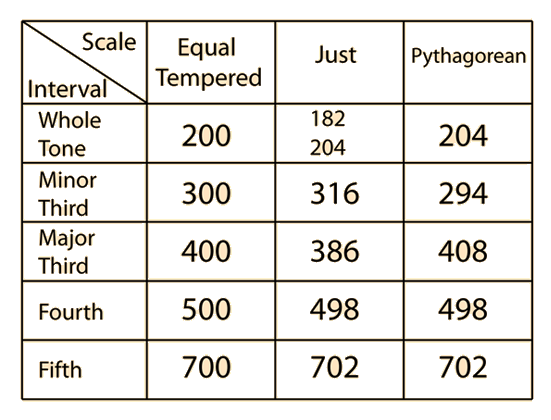The use of cents notation facilitates the comparison of musical intervals in the different systems of temperament. The small-integer-ratio rule for musical consonance is embodied in the just intervals above. The use of 5 cents as the just noticeable difference in pitch helps in the assessment of the magnitudes of the deviations. Using just temperament as the reference, it will be noted that major and minor thirds in equal temperament are the most out of tune with the just intervals. Another useful type of comparison of temperaments is that encountered in the tuning of a major triad.

 Pythagorean intervals
Index

Temperament and musical scales

 HyperPhysics***** Sound R Nave
Go Back